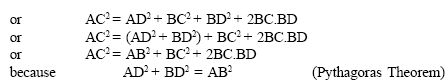Name: ___________________Date:___________________

kwizNET Subscribers, please login to turn off the Ads!
 Email us to get an instant 20% discount on highly effective K-12 Math & English kwizNET Programs!

### High School Mathematics - 28.14 Pythagorean Theorem - Examples

 Theorem: In a right angled triangle, the square on the hypotenuse is equal to the sum of the squares of the other two sides. If the length of the hypotnuse is c and the lengths of other two sides are b and a then according to the pythogorean theorem: c2 = a2 + b2Converse: In a triangle if the square of one side is equal to the squares on the sums of the other two sides, then the angle opposite to the first side is a right angle. To prove: AB2 + BC2 = AC2Example: Prove that the sum of the squares of the sides of a rhombus is equal to the sum of the squares of the diagonals.Directions: Solve the following problems. Also write at least 10 examples of your own.Name: ___________________Date:___________________

### High School Mathematics - 28.14 Pythagorean Theorem - Examples

 Q 1: The sides of a triangle are 50cm, 80cm, 100cm, check if this is a right angled triangle.noyes Q 2: Which of these is a Pythagorean triplet?15, 8, 58, 9, 1313, 12, 5 Q 3: A ladder 20 m long reaches a height of 16m above the ground. Find the distance from the foot of the ladder to the wall.17 m13 m12 m Q 4: Triangle PQR is equilateral. QR = 30 units, B is the midpoint of QA. QA is perpendicular to PR. What is the length of PB? 19.84 cm23 cm24 cm Q 5: The sides of a triangle are 7cm, 24cm, 25cm, check if this is a right angled triangle.yesno Q 6: A person goes 10 m due east and then 30m due north. Find his distance from the starting point.15m12m10m Question 7: This question is available to subscribers only! Question 8: This question is available to subscribers only!

#### Subscription to kwizNET Learning System costs less than \$1 per month & offers the following benefits:

• Unrestricted access to grade appropriate lessons, quizzes, & printable worksheets
• Instant scoring of online quizzes
• Progress tracking and award certificates to keep your student motivated
• Unlimited practice with auto-generated 'WIZ MATH' quizzes
• Child-friendly website with no advertisements

© 2003-2007 kwizNET Learning System LLC. All rights reserved. This material may not be reproduced, displayed, modified or distributed without the express prior written permission of the copyright holder. For permission, contact info@kwizNET.com
For unlimited printable worksheets & more, go to http://www.kwizNET.com.